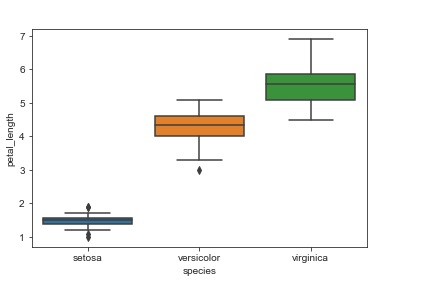# How can box and whisker plot be used to compare the data in different categories in Python Seaborn?

Seaborn library helps in visualizing data. It comes with customized themes and a high level interface.

Scatter plots provide limited information, since they only tell us about the distribution of values within a given category of data. We need to use a different technique if we wish to compare the data present within categories. This is where box plots come into play. It is a way in which the data distribution in the dataset can be understood with the help of quartiles.

It consists of vertical lines that extend from the boxes. These extensions are known as whiskers. These whiskers talks about how the data varies outside the upper and lower quartiles. This is why box plots are also known as whisker plots.

Syntax of boxplot function

seaborn.boxplot(x, y,data,…)

Outliers in the data are plotted as individual points. Here’s the example −

## Example

import pandas as pd
import seaborn as sb
from matplotlib import pyplot as plt
sb.boxplot(x = "species", y = "petal_length", data = my_df)
plt.show()

## Output## Explanation

• The required packages are imported.
• The input data is ‘iris_data’ which is loaded from the scikit learn library.
• This data is stored in a dataframe.
• This data is visualized using the ‘boxplot’ function.
• Here, the dataframe is supplied as parameter.
• Also, the x and y values are specified.
• This data is displayed on the console.

Updated on: 11-Dec-2020

230 Views